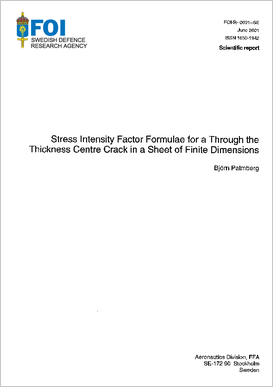# Stress intensity factor formulae for a through the thickness centre crack in a sheet of finite dimensionsAuthors:

Publish date: 2001-01-01

Report number: FOI-R--0091--SE

Pages: 76

Written in: English

## Abstract

Stress intensity factors and stress intensity factor equations for the centre crack in a sheet of finite dimensions have been analyzed. Firstly, a finite width and height correction factor was developed for the sheet loaded i tension along the edge parallel to the crack line. Secondly, the stress intensity factor for the geometry above but with the crack surfaces subjected to a symmetric, partial pressure was obtained using the approximate weight function technique. Thirdly, an equation for this stress intensity factor was developed and verified by finite element analyses. Fourthly, three different stress intensity factor equations for the centre cracked strip subjected to two pairs of symmetrically located splitting forces were studied and compared to the results of finite element computations. It was found that the equation based upon the force-balance method was less accurate compared to the other two equations. Also, in the case of a non symmetric crack surface pressure the equation proposed, based on the force-balance method, showed rather large relative differences compared to the finite element results. Finally, two stress intensity factor equations for the centre cracked strip subjected to a single pair of splitting forces were studied. Compared to finite element results the stress intensity factors obtained by the equation based upon the force-balance method showed large differences. However, more surprisingly, the equation developed by Tada (1973) on basis of the exact mode III stress intensity factor and an asymptotic interpolation showed large differences for the crack tip away from the force pair when compared to the finite element results. The equation is said to have an accuracy better than 1% but the relative differences amounted to more than 75% in some cases.

Share page on social media